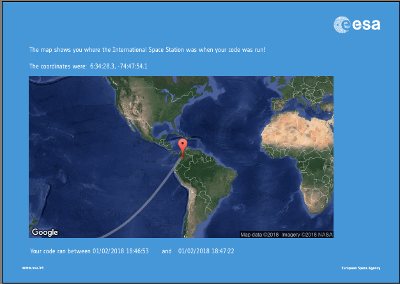Home Family Days Out Raspberry Pi & Electronics Blog

## ESA European Astro Pi Project – Mission Zero – Code that ran on the International Space Station

The ESA European Astro Pi Project – “Mission Zero”, involved school children creating code that actually ran on the International Space Station (ISS).

My son joined with a friend (both aged 9 at the time it was submitted) to create a Python program that ran on the International Space station during February 2018.

Here’s his story:

The AstroPi shown in the video is a 3D printed replica of the Raspberry Pi case that is installed on the Space Station. It includes the Raspberry Pi Sense hat which shows the LED display and includes the temperature sensor.

The project gives a friendly greeting to the astronauts and then displays the temperature in degrees Celsius. If the temperature is warm then it displays a warm picture showing the sun and a smily face, or if it is cold then it displays a snowy scene.

The code has been written in Python. There were some slight modifications used in the video (it included key-presses that allowed the code to be repeated), but the actual code that ran on the Raspberry Pi on the ISS is included below:

``` from sense_hat import SenseHat import time sense = SenseHat() sense.set_rotation(270) red=(255, 0, 0) blue=(10, 0, 255) sense.show_message("Hello, Astronauts", text_colour=red, scroll_speed=0.05) sense.show_message("Have a nice day", text_colour=(0, 0, 255), scroll_speed=0.05) temp = sense.get_temperature() temp = round(sense.get_temperature(), 1) time.sleep(1) sense.show_message("It is " + str(temp) + "Degrees", text_colour=(0, 255, 0), scroll_speed=0.05) g = (0, 255, 0) b = (0, 0, 255) w = (255, 255, 255) y = (255, 255, 0) g = (0, 255, 0) p = (145, 200, 255) hot = [ w, w, w, w, w, y, y, y, w, b, w, b, w, y, y, y, w, b, w, b, w, y, y, y, b, w, w, w, b, w, w, w, b, w, w, w, b, w, w, w, w, b, b, b, w, w, w, w, g, g, g, g, g, g, g, g, g, g, g, g, g, g, g, g ] cold = [ p, p, p, p, p, p, p, p, p, p, p, p, p, w, p, p, p, p, p, w, p, p, p, p, p, p, w, p, p, p, p, p, p, p, p, p, p, w, p, p, p, p, p, p, p, p, p, p, w, w, w, w, w, w, w, w, w, w, w, w, w, w, w, w ] if temp >= 20: sense.set_pixels(hot) time.sleep(2) else: sense.set_pixels(cold) time.sleep(2) ```

The children actually wrote more code initially, but it had to be trimmed down to make it simpler due to the restrictions on the project.

Below are some photos of my son with his certificate and of the 2nd page of the certificate showing that the ISS was in orbit above Columbia at the time the code was run.